• 在图像处理上，导向滤波器（Guided Image Filter）是一种能使图像平滑化的非线性滤波器。与双边滤波器（Bilateral Filter）相同，这个滤波器同样能够在清楚保持图像边界的情况下，达到让图像平滑的效果。 但不同于...
前言
在图像处理上，导向滤波器（Guided Image Filter）是一种能使图像平滑化的非线性滤波器。与双边滤波器（Bilateral Filter）相同，这个滤波器同样能够在清楚保持图像边界的情况下，达到让图像平滑的效果。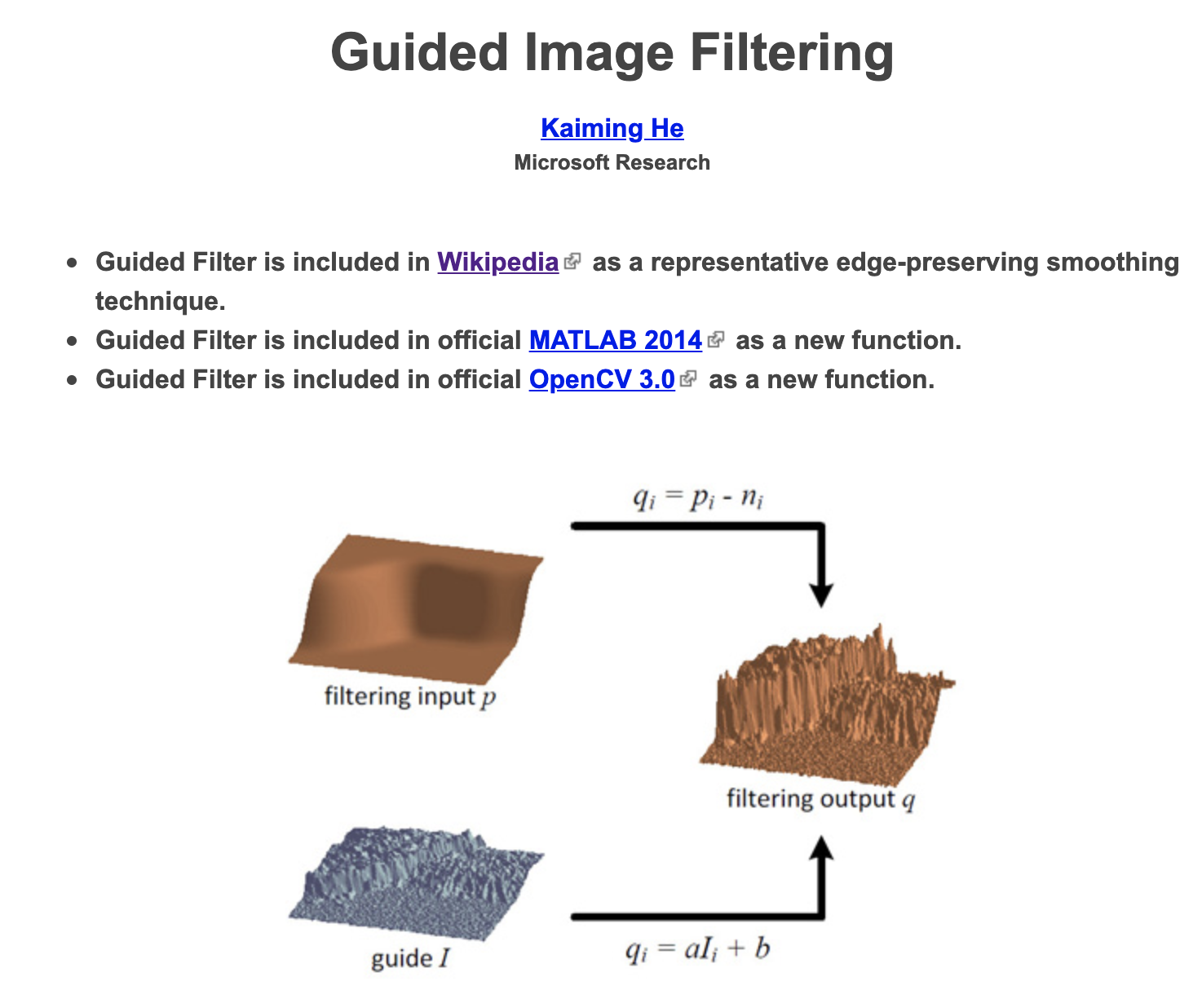但不同于双边滤波器，导向滤波器有两个优点：

首先，双边滤波器有非常大的计算复杂度（O(N^2)），但导向滤波器因为并未用到过于复杂的数学计算，有线性的计算复杂度。

可以说，导向滤波相比双边滤波的两大优势就是速度快和不会有梯度反转。
实际的应用场景除了去噪平滑外，还可以用于细节加强（detail smoothing/enhancement，如“羽化”）、HDR compression、image matting/feathering、haze removal（去雾）、joint upsampling、深度图修整等功能。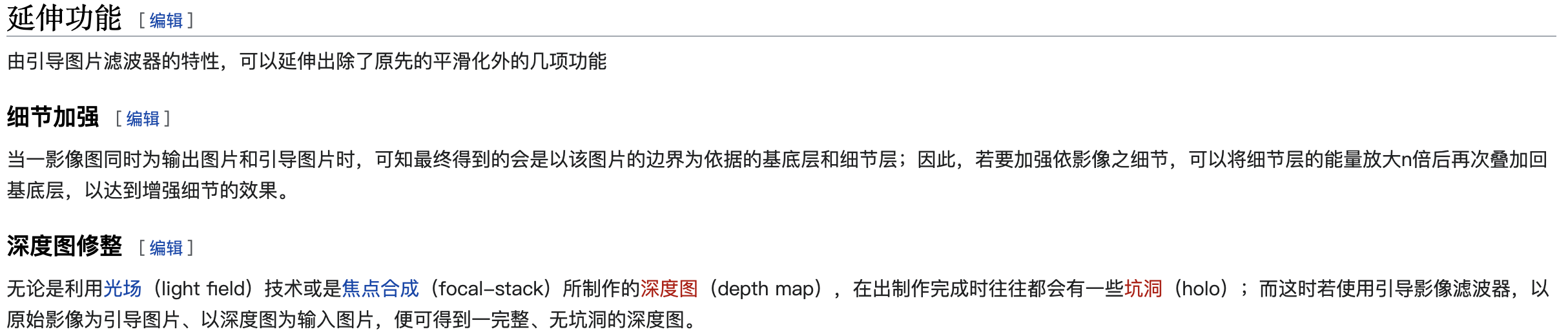原理
为了达到图像平滑去噪效果，首先定义输出的结果图是输入图减去噪声后的结果。同时，为了让输出图保持引导图的边界，将输出图定为引导图的线性组合。
可以说，导向滤波核心原理是假设导向图I与滤波结果输出图q符合局部（以像素$k$为中心的$w_k$窗口内）线性模型：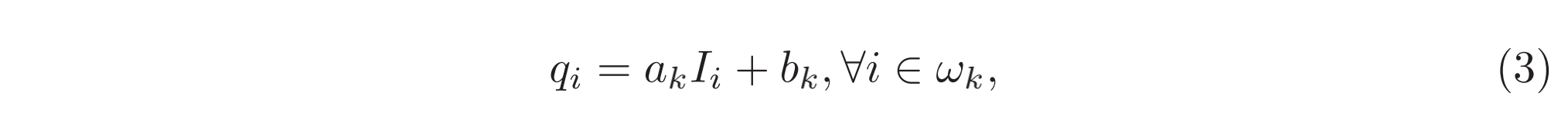局部线性模型（local linear model）保证了结果图与导向图的edge一致（$∇q = a∇I$）。
为了得到线性系数，需要构建方程求解。论文采用的是最小化输出q与输入图p之间的差异，即最小化窗口内的代价函数：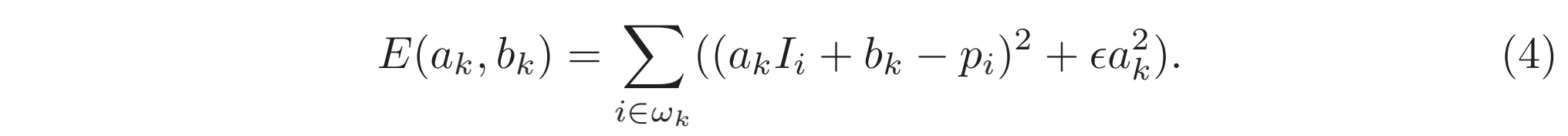其中 $\epsilon$ 是防止 $a_k$ 过大的正则化参数。
方程的解可以根据 linear regression 求得，细节见参考资料或中推导：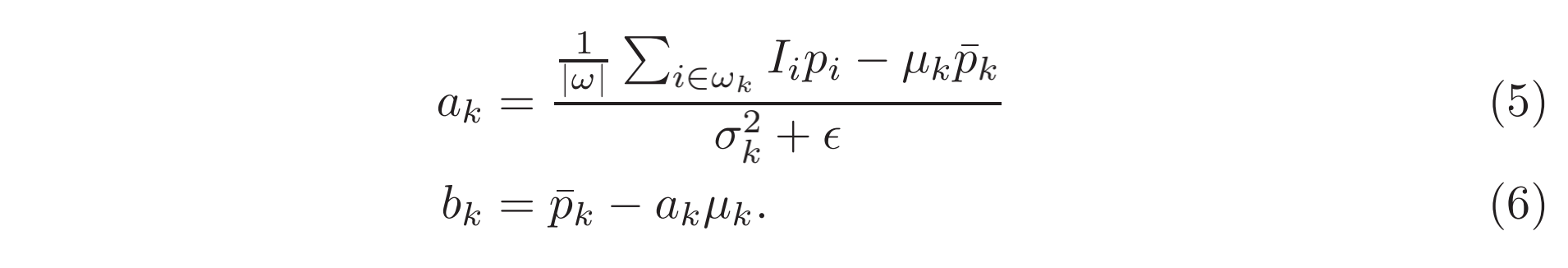其中，$\mu_k$和$\sigma^2_k$是导向图I在窗口$w_k$内的均值和方差，$|w|$是窗口$w_k$内的像素数目，$\overline{p}_k=\frac{1}{|w|}\sum_{i\in w_k}{p_i}$是窗口$w_k$内的均值。
基本上，根据得到的$a_k$和$b_k$就可以计算得出窗口$w_k$内的每一个$q_i$。但是进一步考虑，由于每一个像素不一定只被一个窗口$w_k$所包含，例如九宫格情况下中心像素点就被9个3x3的$w_k$窗口包含。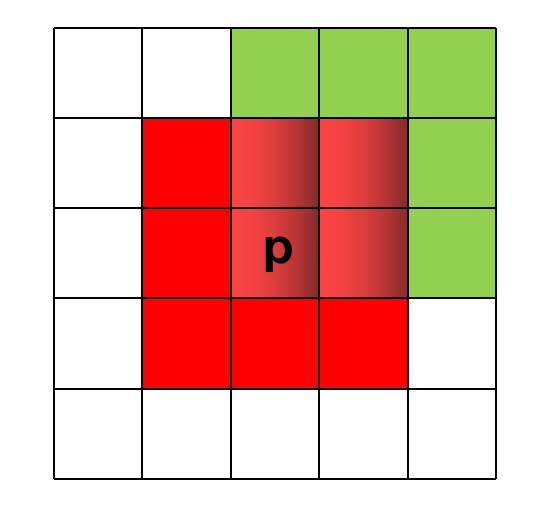所以最简单的方式则是对这9个$w_k$窗口得到的$q_i$做一个加权平均，得到的最终$q_i$才是真正的结果值。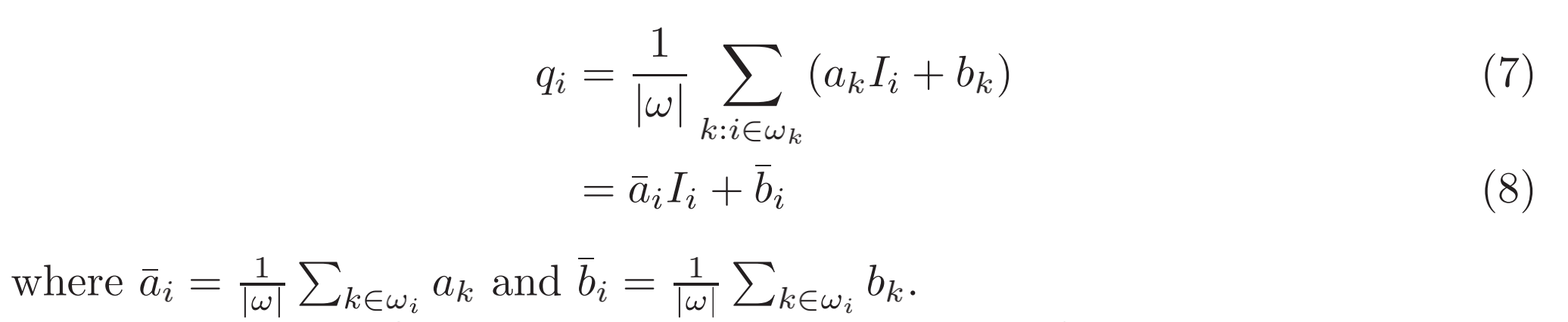经过对所有$q_i$的加权平均（实际上用的是均值滤波），$∇q$不再是 $∇I$线性关系。但是由于$(\overline a_i, \overline b_i)$是经过均值滤波得到，在导向图的强边界处，输出图的梯度会比导向图小。这种情况下可以认为$∇q \approx \overline a∇I$，表示导向图I边界的强变化还能被输出图q维持。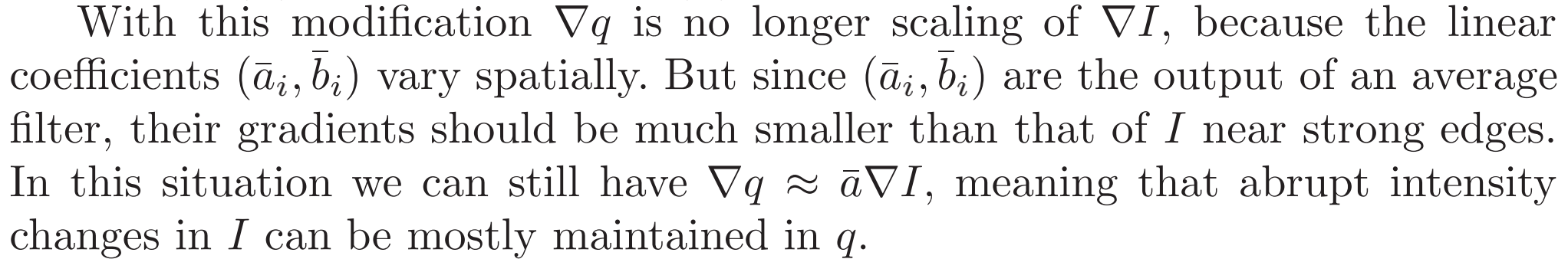算法伪码如下：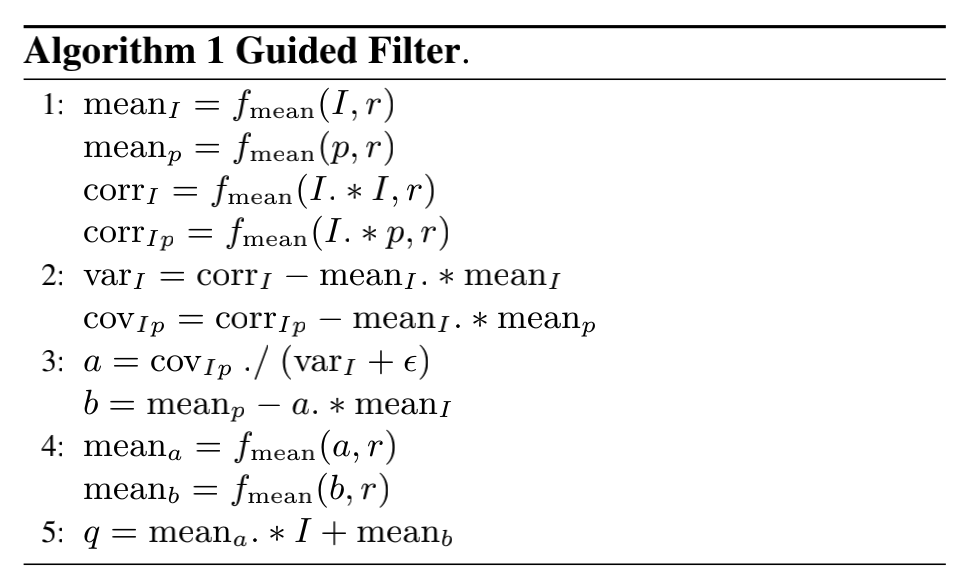其中，$f_{mean}(·, r)$ 是半径为r的均值滤波器。
而方差和协方差定义如下：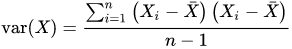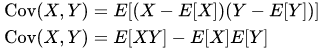对式子 (5) 进行变换，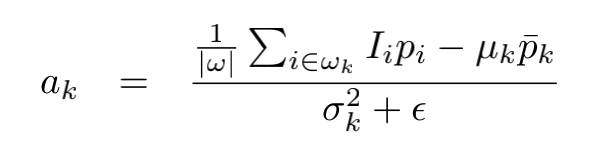则可以得到算法伪码中的：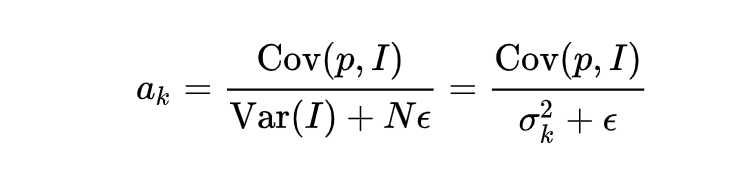特别说明：
通过参数$\epsilon$ 定义什么是“平坦区块(patch)”或“高变化区块”。若一个区块的方差远低于参数$\epsilon$ ，其通过滤波器后将被平滑；反之，方差远高于$\epsilon$的区块将被视为边界而被保留。
双边滤波中的范围方差(range variance)参数$\sigma _{r}^{2}$的功能和导向滤波的$\epsilon$相似。它们都定义了什么样的区块应该被平滑，而什么样的区块应该被保留。
实现
OpenCV中对导向滤波有CPU实现。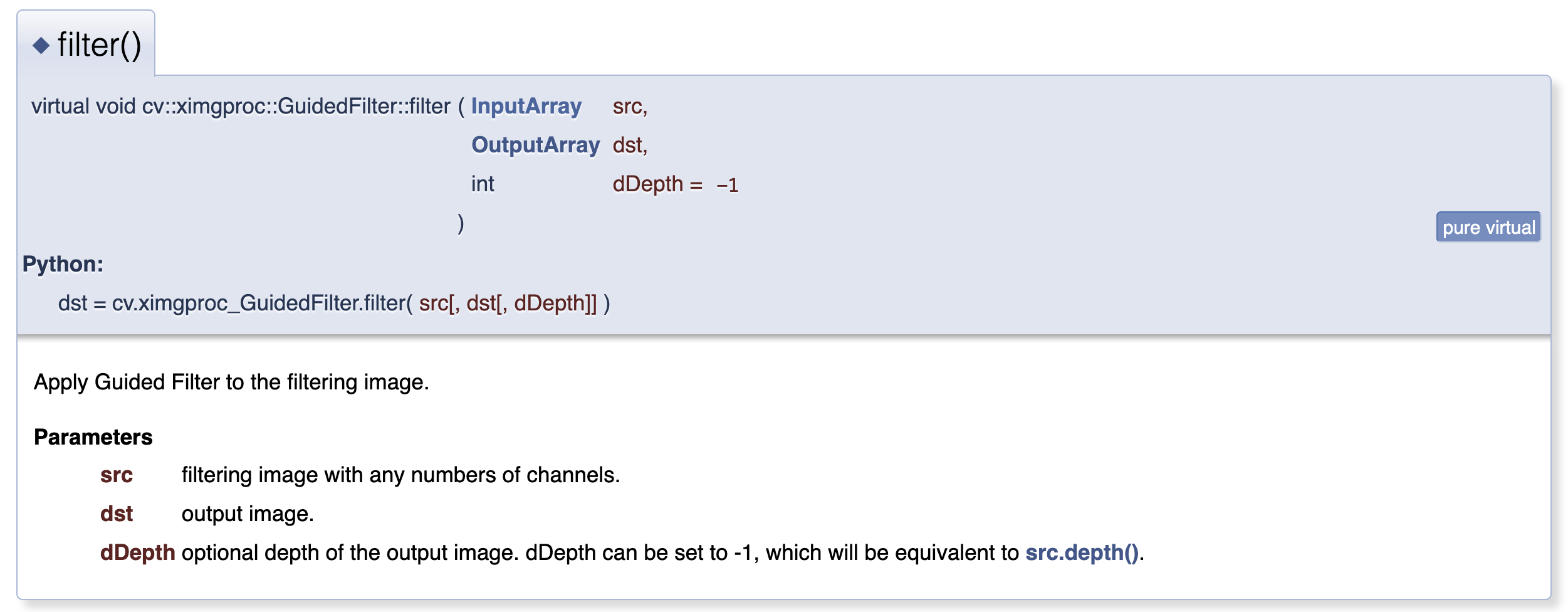核心代码如下：
void GuidedFilterImpl::filter(InputArray src, OutputArray dst, int dDepth /*= -1*/)
{
CV_Assert( !src.empty() && (src.depth() == CV_32F || src.depth() == CV_8U) );
if (src.rows() != h || src.cols() != w)
{
CV_Error(Error::StsBadSize, "Size of filtering image must be equal to size of guide image");
return;
}

if (dDepth == -1) dDepth = src.depth();
int srcCnNum = src.channels();

vector<Mat> srcCn(srcCnNum);
vector<Mat>& srcCnMean = srcCn;
split(src, srcCn);

if (src.depth() != CV_32F)
{
parConvertToWorkType(srcCn, srcCn);
}

vector<vector<Mat> > covSrcGuide(srcCnNum);
computeCovGuideAndSrc(srcCn, srcCnMean, covSrcGuide);

vector<vector<Mat> > alpha(srcCnNum);
for (int si = 0; si < srcCnNum; si++)
{
alpha[si].resize(gCnNum);
for (int gi = 0; gi < gCnNum; gi++)
alpha[si][gi].create(h, w, CV_32FC1);
}
runParBody(ComputeAlpha_ParBody(*this, alpha, covSrcGuide));
covSrcGuide.clear();

vector<Mat>& beta = srcCnMean;
runParBody(ComputeBeta_ParBody(*this, alpha, srcCnMean, beta));

parMeanFilter(beta, beta);
parMeanFilter(alpha, alpha);

runParBody(ApplyTransform_ParBody(*this, alpha, beta));
if (dDepth != CV_32F)
{
for (int i = 0; i < srcCnNum; i++)
beta[i].convertTo(beta[i], dDepth);
}
merge(beta, dst);
}


具体文件参考GitHub的OpenCV Contrib包实现。
GPU版导向滤波实现参考GitHub - TracelessLe/pybind11_guidedfilter_cuda。
cv::cuda::GpuMat GuidedFilterMono::filterSingleChannel(const cv::cuda::GpuMat &p, cv::cuda::Stream &stream) const {
cv::cuda::GpuMat mean_p, mean_Ip, cov_Ip;
box_filter->apply(p, mean_p, stream);
cv::cuda::multiply(I, p, mean_Ip, 1, -1, stream);
box_filter->apply(mean_Ip, mean_Ip, stream);
cv::cuda::multiply(mean_I, mean_p, cov_Ip, 1, -1, stream);
cv::cuda::subtract(mean_Ip,
cov_Ip,
cov_Ip,
cv::noArray(),
-1,
stream); // this is the covariance of (I, p) in each local patch.

cv::cuda::GpuMat a, b;
cv::cuda::add(var_I, cv::Scalar(eps), a, cv::noArray(), -1, stream);
cv::cuda::divide(cov_Ip, a, a, 1, -1, stream); // Eqn. (5) in the paper;

cv::cuda::multiply(a, mean_I, b, 1, -1, stream);
cv::cuda::subtract(mean_p, b, b, cv::noArray(), -1, stream); // Eqn. (6) in the paper;

box_filter->apply(a, a, stream);
box_filter->apply(b, b, stream);

cv::cuda::multiply(a, I, a, 1, -1, stream);
cv::cuda::add(a, b, a, cv::noArray(), -1, stream);

return a;
}

扩展讨论
（1）相比双边滤波，导向滤波有速度快和避免梯度反转等优势。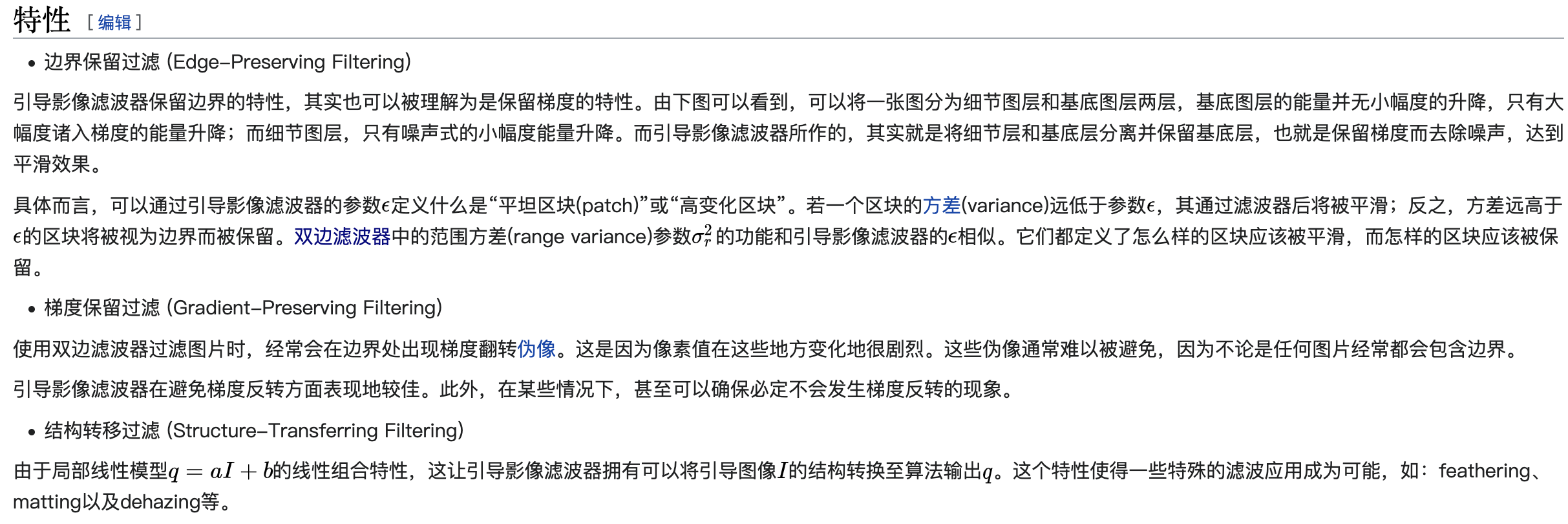（2）基于原始的导向滤波算法引入resize得到的Fast导向滤波能够将时间复杂度从O(N)降到O(N / r^2)，同时保证滤波结果图像质量损失不大。其中r是resize（或称之为scale）的倍数。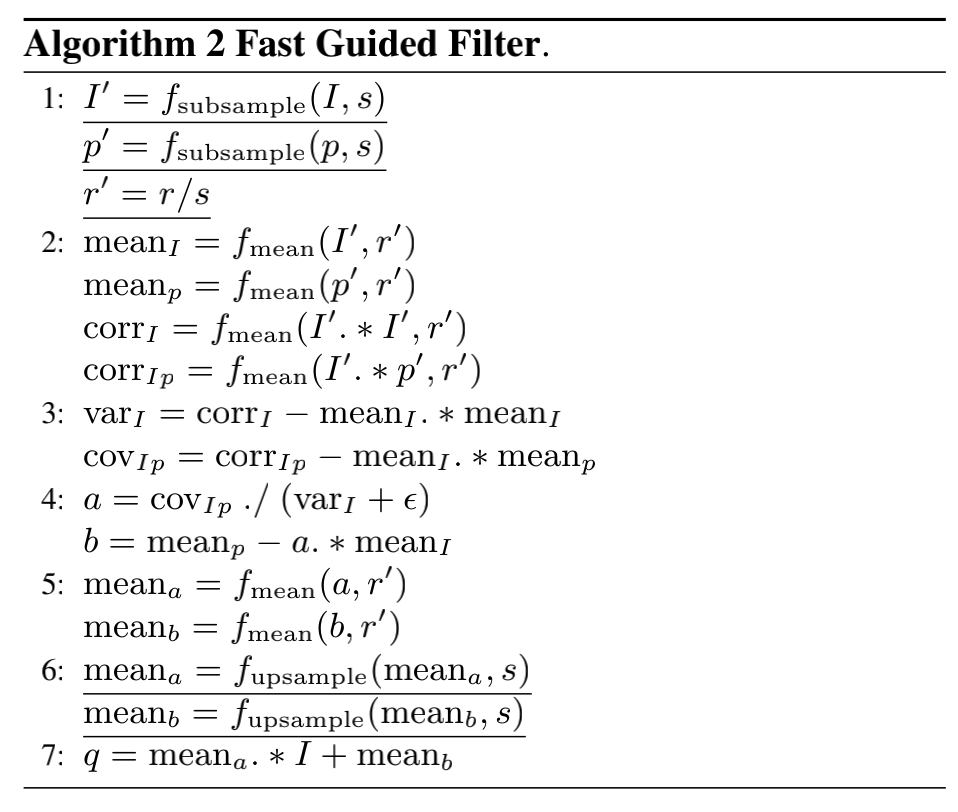参考资料
 wikipedia - Edge-preserving smoothing
 维基百科 - 引导影像滤波器
 Guided Image Filtering - Kaiming He
 GitHub - opencv_contrib/modules/ximgproc/src/guided_filter.cpp
 OpenCV Docs - GuidedFilter
 知乎 - 导向滤波原理（Guided Filter）
 知乎 - 引导滤波guideFilter原理推导与实验
 维基百科 - 方差
 维基百科 - 协方差
  知网 - 引导滤波算法的CUDA加速实现
 豆丁网 - 引导滤波算法的CUDA加速实现
 GitHub - acstacey/GLFCV/src/guidedfilter.cpp
 GitHub - xxxzhou/oeip/oeip-win-cuda/GuidedFilterLayer.cpp
 cnblogs - CUDA加opencv复现导向滤波算法
 GitHub - foowaa/3DVisionUnit/GuidedFitlerOptimzation_CUDA/GuidedFilter.cu
 GitHub - TracelessLe/pybind11_guidedfilter_cuda
 csdn - 双边滤波原理浅析
 导向滤波 Guided Image Filtering


展开全文计算机视觉 opencv
• 当引导图比较陡峭时，输出图像应随着引导图变化变化，当引导图较为缓和时，输出图像应接近输入图像。 二、原理 引导滤波的思想是： 认为输出图像与引导图像在 小范围 内是 线性关系 ，将起伏不定的连续像素看成一...
一、性质
引导滤波的特性是：
当引导图比较陡峭时，输出图像应随着引导图变化而变化，当引导图较为缓和时，输出图像应接近输入图像。
二、原理
引导滤波的思想是：
认为输出图像与引导图像在 小范围 内是 线性关系 ，将起伏不定的连续像素看成一段段的小线性函数，每一小段的公式如下：
$q_{i}=a_{k}I_{i}+b_{k}$
其中i是像素坐标，I为引导图像素值，q为线性函数拟合的输出图像，ak、bk是线性函数的两个参数，我们将这个线性函数的适用范围记为k。
也就是说，输出图像要跟着导向图的趋势走，在一个小范围内，如果导向图是平坦的，那么a趋近于0，输出图像q应等于b，如果导向图较为陡峭，a很大，则输出图像q应接近a*I
（当导向图为原图时，a*I相当于原图乘以一个系数，当a=1、b=0，那直接就是原图）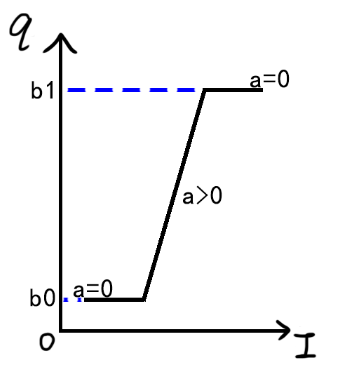三、损失函数
由上一节的线性公式得知，如果变化是陡峭的，斜率是大的，那系数a可以很容易通过初一的知识得到：
$a=\frac{\Delta q }{\Delta I }$
但如果变化是平坦的，b又该如何得到呢？这时候就该原图登场了，由第一节的概念可知，平坦时输出图像应接近原图，因此可写作下面的式子：
$q_{i}-p_{i}=n_{i}$
这里n代表原图与输出的误差。
为了成功还原原图，我们需要最小化误差n，因此常用的损失函数如下（令E最小）：
$E(a_{k},b_{k})=\sum_{i\in w_{k}}^{}(q_{i}-p_{i})^{2}$
这里的wk代表滤波窗口的范围，将q展开：
$E(a_{k},b_{k})=\sum_{i\in w_{k}}^{}(a_{k}I_{i}+b_{k}-p_{i})^{2}$
分别令E对a和b求导，得到a与b的解之后，我们的线性函数能够较为完美的拟合原图，以至于当引导图为原图时，输出完全就是原图。如果最终的结果是这样，那就很难做到保边缘滤波了。因为已经完全没有了滤波。
通常，我们还是希望能够控制这种拟合的程度，在机器学习中，为了防止过拟合，需要在损失函数中引入正则化，在这里也可以同样操作：
$E(a_{k},b_{k})=\sum_{i\in w_{k}}^{}((a_{k}I_{i}+b_{k}-p_{i})+\epsilon a_{k}^{2})^{2}$
其中$\epsilon$叫做正则化系数，可以调节拟合程度，当取值为0时，平坦区域就更接近原图。
用自变量前面的参数来防止过拟合的原因，大体可以解释如下：

拟合过程中通常都倾向于让权值尽可能小，最后构造一个所有参数都比较小的模型。因为一般认为参数值小的模型比较简单，能适应不同的数据集，也在一定程度上避免了过拟合现象。可以设想一下对于一个线性回归方程，若参数很大，那么只要数据偏移一点点，就会对结果造成很大的影响；但如果参数足够小，数据偏移得多一点也不会对结果造成什么影响，专业一点的说法是『抗扰动能力强』。
https://blog.csdn.net/jinping_shi/article/details/52433975
不懂就记住好了。。

对加入了正则化项的公式求ak、bk的偏导，令导数为0，可求得：
$b_{k}=\bar{p_{k}}-a_{k}\bar{I_{k}}$

$a_{k}=\frac{\overline{p_{k}I_{k}}-\overline{p_{k}}\overline{I_{k}}}{\sigma ^{2}+\epsilon }$
公式中出现了均值和方差，这都是在求导过程中凑出来的。
至此，输出图像可直接由$q_{i}=a_{k}I_{i}+b_{k}$算出。
四、保边缘滤波
当引导图为原图时，导向滤波是一个很好的保边缘滤波器，怎么理解呢？要从ak和bk的公式说起。
根据第三节最后的公式，我们令Ik等于pk，可得：
$a_{k}=\frac{\overline{p_{k}^{2}} - \overline{p_{k}}^{2}}{\sigma ^{2}+\epsilon } =\frac{\sigma ^{2}}{\sigma ^{2}+\epsilon }$

$b_{k}=(1-a_{k})\overline{p_{k}}$
请出关键公式(I已经替换为p)：
$q_{i}=a_{k}p_{i}+b_{k}$
在图像的边缘处，方差较大，ak趋于1，bk趋于0，输出图像等于输入图像，实现保边缘
在图像的平坦/纹理处，方差较小，ak较小，输出更接近bk，而bk趋于图像均值$\overline{p_{k}}$，实现均值滤波
滤波的程度则由$\epsilon$控制，其为0时，输出永远等于输入图像，与第三节的结论相同。


展开全文图像处理
• 而e的作用就是界定什么是变化大，什么是变化小。在窗口大小不变的情况下，随着e的增大，滤波效果越明显。 在滤波效果上，引导滤波和双边滤波差不多，在一些细节上，引导滤波较好。引导滤波最大的优势在于，可以写出...
一、双边滤波双边滤波很有名，使用广泛，简单的说就是一种同时考虑了像素空间差异与强度差异的滤波器，因此具有保持图像边缘的特性。先看看我们熟悉的高斯滤波器：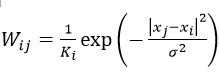其中W是权重，i和j是像素索引，K是归一化常量。公式中可以看出，权重只和像素之间的空间距离有关系，无论图像的内容是什么，都有相同的滤波效果。再来看看双边滤波器，它只是在原有高斯函数的基础上加了一项，如下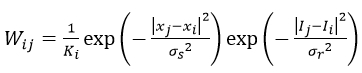其中 I 是像素的强度值，所以在强度差距大的地方（边缘），权重会减小，滤波效应也就变小。总体而言，在像素强度变换不大的区域，双边滤波有类似于高斯滤波的效果，而在图像边缘等强度梯度较大的地方，可以保持梯度。二、引导滤波引导滤波是近三年才出现的滤波技术，知道的人还不多。它与双边滤波最大的相似之处，就是同样具有保持边缘特性。在引导滤波的定义中，用到了局部线性模型，至于该模型，可以暂时用下图简单的理解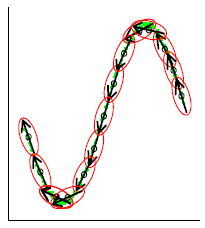该模型认为，某函数上一点与其邻近部分的点成线性关系，一个复杂的函数就可以用很多局部的线性函数来表示，当需要求该函数上某一点的值时，只需计算所有包含该点的线性函数的值并做平均即可。这种模型，在表示非解析函数上，非常有用。同理，我们可以认为图像是一个二维函数，而且没法写出解析表达式，因此我们假设该函数的输出与输入在一个二维窗口内满足线性关系，如下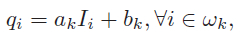其中，q是输出像素的值，I是输入图像的值，i和k是像素索引，a和b是当窗口中心位于k时该线性函数的系数。其实，输入图像不一定是待滤波的图像本身，也可以是其他图像即引导图像，这也是为何称为引导滤波的原因。对上式两边取梯度，可以得到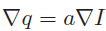即当输入图像I有梯度时，输出q也有类似的梯度，现在可以解释为什么引导滤波有边缘保持特性了。下一步是求出线性函数的系数，也就是线性回归，即希望拟合函数的输出值与真实值p之间的差距最小，也就是让下式最小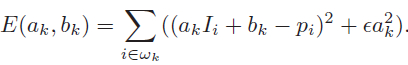这里p只能是待滤波图像，并不像I那样可以是其他图像。同时，a之前的系数（以后都写为e）用于防止求得的a过大，也是调节滤波器滤波效果的重要参数。通过最小二乘法，我们可以得到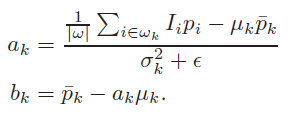其中，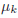是I在窗口w_k中的平均值，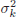是I在窗口w_k中的方差，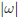是窗口w_k中像素的数量，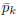是待滤波图像p在窗口w_k中的均值。在计算每个窗口的线性系数时，我们可以发现一个像素会被多个窗口包含，也就是说，每个像素都由多个线性函数所描述。因此，如之前所说，要具体求某一点的输出值时，只需将所有包含该点的线性函数值平均即可，如下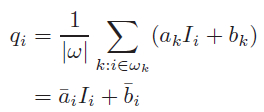这里，w_k是所有包含像素i的窗口，k是其中心位置。当把引导滤波用作边缘保持滤波器时，往往有 I = p ，如果e=0，显然a=1, b=0是E(a,b)为最小值的解，从上式可以看出，这时的滤波器没有任何作用，将输入原封不动的输出。如果e>0，在像素强度变化小的区域（或单色区域），有a近似于（或等于）0，而b近似于（或等于），即做了一个加权均值滤波；而在变化大的区域，a近似于1，b近似于0，对图像的滤波效果很弱，有助于保持边缘。而e的作用就是界定什么是变化大，什么是变化小。在窗口大小不变的情况下，随着e的增大，滤波效果越明显。在滤波效果上，引导滤波和双边滤波差不多，在一些细节上，引导滤波较好。引导滤波最大的优势在于，可以写出时间复杂度与窗口大小无关的算法（打算在之后的文章中讨论），因此在使用大窗口处理图片时，其效率更高。三、opencv实现Mat guidedfilter(Mat &img, Mat &dst, int r, double esp);
int main()
{
Mat image, dst_image, temp_image,P,Q;
vector<Mat>src, dst(3);
split(image, src);
for (int i = 0; i < 3; i++)
{
src[i].convertTo(temp_image, CV_64FC1,1.0/255.0);
P = temp_image.clone();
dst_image = guidedfilter(temp_image, P, 5, 0.01);
dst_image.convertTo(dst_image, CV_8UC1, 255.0);
dst[i] = dst_image;
}
merge(dst, Q);

waitKey();
return 0;
}
Mat guidedfilter(Mat &img, Mat &dst, int r, double esp)
{
int row = img.rows;
int col = img.cols;
img.convertTo(img, CV_64FC1);
dst.convertTo(dst, CV_64FC1);
Mat boxResult,mean_I,mean_P,result,mean_IP, cov_IP, mean_II, var_I;
Mat a, b, mean_a, mean_b;
boxFilter(Mat::ones(Size(row,col), img.type()), boxResult, CV_64FC1, Size(r, r));//计算均值N
boxFilter(img, mean_I, CV_64FC1, Size(r, r));
mean_I = mean_I / boxResult;//计算导向均值mean_I
boxFilter(dst, mean_P, CV_64FC1, Size(r, r));
mean_P = mean_P / boxResult;//计算原始均值mean_P
boxFilter(img.mul(dst), mean_IP, CV_64FC1, Size(r, r));
mean_IP = mean_IP / boxResult;//计算互相关均值mean_IP
cov_IP = mean_IP - mean_I.mul(mean_P);
boxFilter(img.mul(img), mean_II, CV_64FC1, Size(r, r));
mean_II = mean_II / boxResult;//计算自相关均值mean_II
var_I = mean_II - mean_I.mul(mean_I);//计算var_I
a = cov_IP / (var_I + esp);//计算相关系数
b = mean_P - a.mul(mean_I);
boxFilter(a, mean_a, CV_64FC1, Size(r, r));
boxFilter(b, mean_b, CV_64FC1, Size(r, r));
mean_a = mean_a / boxResult;//计算mean_a
mean_b = mean_b / boxResult;//计算mean_b
result = mean_a.mul(img) + mean_b;
return result;
}结果如下：听说导向滤波还有去雾的作用，感兴趣的大家可以看看。
展开全文• 1概述随着煤矿采煤机械化水平的不断提高,采煤机已成为综采工作面主要的...由于受煤层地质等因素的影响,综采工作面会出现断层、褶曲、煤层起伏变化等异常地段。回采期间采煤机震动大,采煤机的支撑点———导向滑靴损害
• ## OpenCV—Python 导向滤波

千次阅读 多人点赞 2018-11-19 15:23:13
一、导向滤波原理 导向滤波是使用导向图像作为滤波内容图像，在导向图像上实现局部线性函数表达，实现各种不同的线性变换，输出变形之后的导向滤波图像。根据需要，导向图像可以跟输入图像不同或者一致。 公式及...
一、导向滤波原理
导向滤波是使用导向图像作为滤波内容图像，在导向图像上实现局部线性函数表达，实现各种不同的线性变换，输出变形之后的导向滤波图像。根据需要，导向图像可以跟输入图像不同或者一致。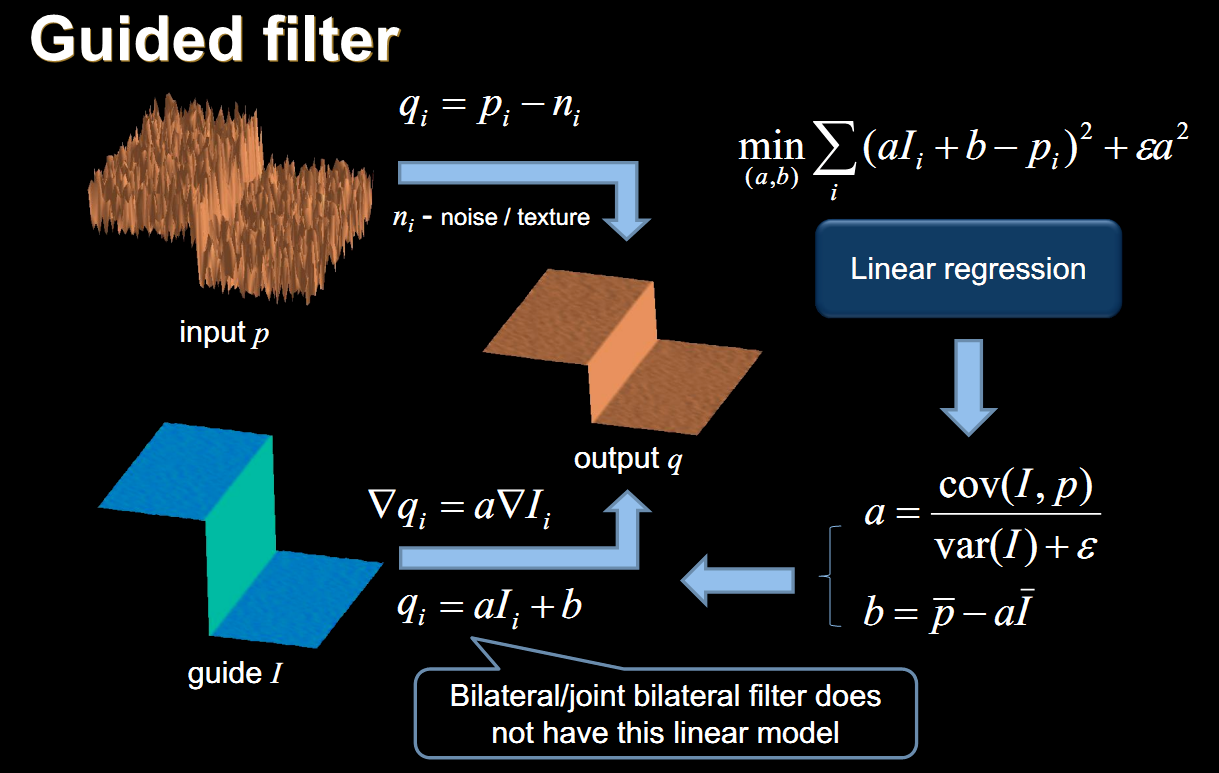公式及推导
假设 $I$ 是导向图像、$p$ 是输入图像、$q$ 是导向滤波输出图像；导向滤波是作为局部线性模型描述 导向图像 $I$ 与 输出图像 $q$ 之间的关系。
对于任意像素 $\rm I$ 来说，$\omega_k$窗口下的线性变换可以表述如下：
$q_i = a_k I_i + b_k$ 其中 $(a_k,b_k)$是窗口 $\omega_k$ 范围内的参数常量。
为了寻找线性相关性，窗口 $\omega_k$ 定义的损失函数为：
$E(a_k,b_k) = \sum_{i \in \omega_k} ((a_k I_i + b_k-p_i)^2+\epsilon a_k^2)$
其中：
$\omega_k$是对 $a_k$ 值过大时侯的正则化补偿。
$\varepsilon a_k^2$ 是抑制 $a_k$ 值过大的。
$\varepsilon$ 是调整图的模糊程度与边缘检测精度的参数。
如果导向图 $\rm I$ 没有边缘信息，输出均值模糊结果；
如果导向图 $\rm I$ 包含边缘信息，边缘信息则迁移到输出图像中实现边缘保留滤波；
上述的损失函数可以被看成一个线性回归问题，其中两个参数的求解如下：

$a_k = \frac{\frac{1}{\omega_k}\sum_{i \in \omega_k} I_iP_i-\mu_k \bar{p}_k}{\sigma_k^2 + \epsilon}$

$b_k = \bar{p}_k - a_k\mu_k$

$\mu_k$ 与 $\sigma_k^2$ 是导向图在 $\omega_k$ 窗口大小均值与方差

$|\omega|$表示窗口内像素总数

$\bar{p}_k = \frac{1}{|\omega|}\sum_{i \in \omega_k}p_i$ 在 $\omega_k$ 窗口内输入图像像素均值

使用线性相关参数 $(a_k,b_k)$，滤波输出图像就可以通过$q_i = a_k I_i + b_k$ 线性模型得到。
针对不同的窗口大小我们就会得到不同的 $q_i$ 值，所以通过它的均值作为最终的输出结果：
$q_i = \frac{1}{\omega_k}\sum_{i \in \omega_k}(a_k I_i + b_k) = \bar{a}_iI_i+\bar{b}_i$
最终导向滤波公式为：
$\bar{a}_，\bar{b}_i$ 是所有像素点 $i$ 上重叠窗口相关因子的均值。
导向滤波算法实现的一般步骤为：

读取导向图像 $\rm I$ 与 输入图像 $\rm P$
积分图计算 $\rm I$ 的均值与方差、输入图像 $\rm P$的均值、 $\rm I$ 与 $\rm P$的乘积 $\rm IP$
计算线性相关因子 $\rm a$ 与 $\rm b$
$a=(IP-I_{mean}P_{mean})/(I_{Var} +\epsilon )$
$b=P_{mean}-\textrm{a}I_{mean}$
计算a与b的均值
使用均值得到导向滤波结果 $Q = a_{mean}*I+b_{mean}$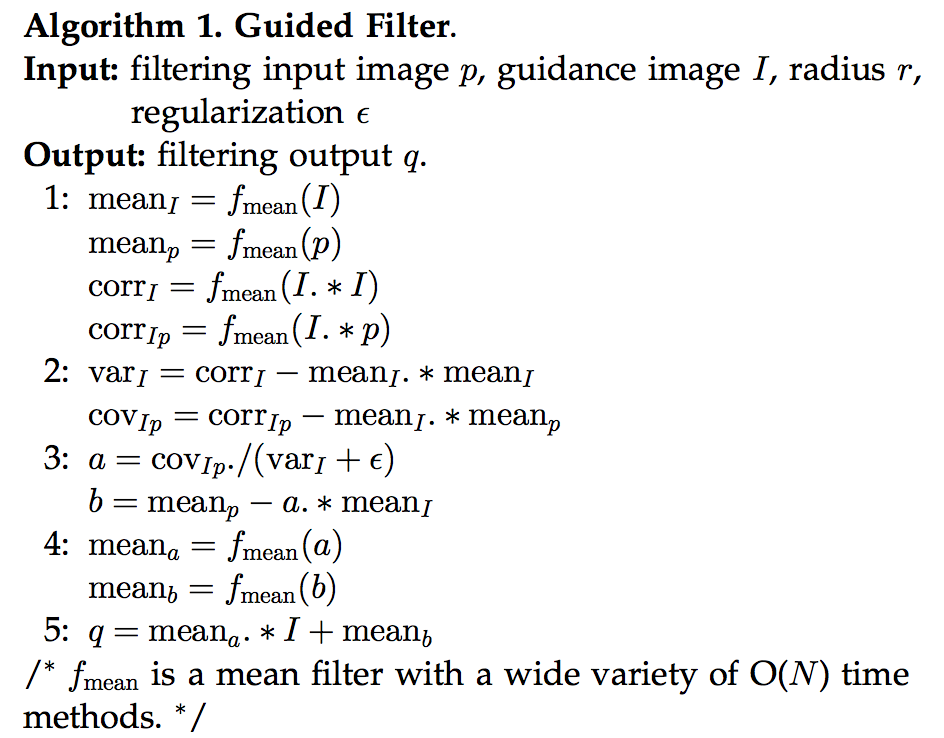导向滤波最常用四个功能是：

边缘保留滤波
图像去噪声
图像边缘羽化
图像增强（对比度）

import numpy as np
import cv2

def guideFilter(I, p, winSize, eps):

mean_I = cv2.blur(I, winSize)      # I的均值平滑
mean_p = cv2.blur(p, winSize)      # p的均值平滑

mean_II = cv2.blur(I * I, winSize) # I*I的均值平滑
mean_Ip = cv2.blur(I * p, winSize) # I*p的均值平滑

var_I = mean_II - mean_I * mean_I  # 方差
cov_Ip = mean_Ip - mean_I * mean_p # 协方差

a = cov_Ip / (var_I + eps)         # 相关因子a
b = mean_p - a * mean_I            # 相关因子b

mean_a = cv2.blur(a, winSize)      # 对a进行均值平滑
mean_b = cv2.blur(b, winSize)      # 对b进行均值平滑

q = mean_a * I + mean_b
return q

if __name__ == '__main__':
eps = 0.01
winSize = (5,5)
image = cv2.resize(image, None,fx=0.7, fy=0.7, interpolation=cv2.INTER_CUBIC)
I = image/255.0        #将图像归一化
p =I
guideFilter_img = guideFilter(I, p, winSize, eps)

# 保存导向滤波结果
guideFilter_img  = guideFilter_img  * 255
guideFilter_img [guideFilter_img  > 255] = 255
guideFilter_img  = np.round(guideFilter_img )
guideFilter_img  = guideFilter_img.astype(np.uint8)
cv2.imshow("image",image)
cv2.imshow("winSize_5", guideFilter_img )
cv2.waitKey(0)
cv2.destroyAllWindows()

当 $I=p$ 时，导向滤波就变成了边缘保持的滤波操作，此时原来求出的$a$ 和 $b$ 的表达式就变成了：
$a_k=\frac{σ_k^2}{σ_k^2+ϵ}$
$b_k=(1−a_k)μ_k$
考虑两种情况：

情况1：高方差区域，即表示图像 $I$ 在窗口 $w_k$ 中变化比较大，此时我们有$σ_k^2>>ϵ$，于是有$a_k≈1$和$b_k≈0$。
情况2：平滑区域（方差不大），即图像 $I$ 在窗口 $w_k$ 中基本保持固定，此时有$σ_k^2<<ϵ$，于是有$a_k≈0$和$b_k≈μ_k$ 。

也就是说在方差比较大的区域，保持值不变，在平滑区域，使用临近像素平均（也就退化为普通均值滤波）。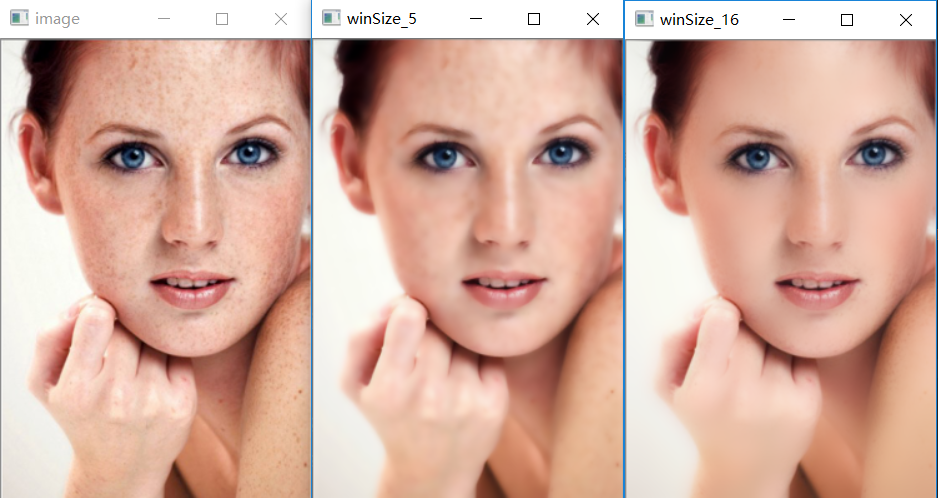快速导向滤波
通过下采样减少像素点，计算 $\rm mean_a$ & $\rm mean_b$ 后进行上采样恢复到原有的尺寸大小。
假设缩放比例为s,那么缩小后像素点的个数为$N/s^2，$那么时间复杂度变为$O(N/s^2)$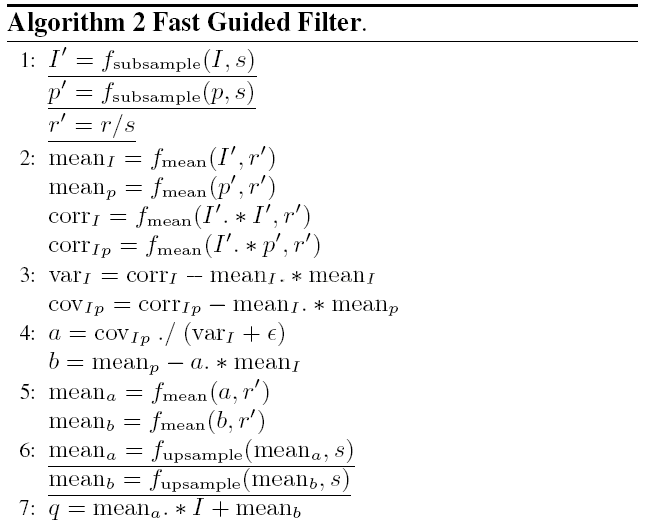import cv2
import numpy as np

def guideFilter(I, p, winSize, eps, s):
# 输入图像的高、宽
h, w = I.shape[:2]

# 缩小图像
size = (int(round(w * s)), int(round(h * s)))
small_I = cv2.resize(I, size, interpolation=cv2.INTER_CUBIC)
small_p = cv2.resize(I, size, interpolation=cv2.INTER_CUBIC)

# 缩小滑动窗口
X = winSize
small_winSize = (int(round(X * s)), int(round(X * s)))

# I的均值平滑 p的均值平滑
mean_small_I = cv2.blur(small_I, small_winSize)
mean_small_p = cv2.blur(small_p, small_winSize)

# I*I和I*p的均值平滑
mean_small_II = cv2.blur(small_I * small_I, small_winSize)
mean_small_Ip = cv2.blur(small_I * small_p, small_winSize)

# 方差、协方差
var_small_I = mean_small_II - mean_small_I * mean_small_I
cov_small_Ip = mean_small_Ip - mean_small_I * mean_small_p

small_a = cov_small_Ip / (var_small_I + eps)
small_b = mean_small_p - small_a * mean_small_I

# 对a、b进行均值平滑
mean_small_a = cv2.blur(small_a, small_winSize)
mean_small_b = cv2.blur(small_b, small_winSize)

# 放大
size1 = (w, h)
mean_a = cv2.resize(mean_small_a, size1, interpolation=cv2.INTER_LINEAR)
mean_b = cv2.resize(mean_small_b, size1, interpolation=cv2.INTER_LINEAR)

q = mean_a * I + mean_b

return q

if __name__ == '__main__':
eps = 0.01
winSize = (16,16)       #类似卷积核（数字越大，磨皮效果越好）
image = cv2.resize(image,None,fx=0.8,fy=0.8,interpolation=cv2.INTER_CUBIC)
I = image/255.0       #将图像归一化
p =I
s = 3 #步长
guideFilter_img = guideFilter(I, p, winSize, eps,s)

# 保存导向滤波结果
guideFilter_img = guideFilter_img  * 255         #(0,1)->(0,255)
guideFilter_img[guideFilter_img  > 255] = 255    #防止像素溢出
guideFilter_img = np.round(guideFilter_img )
guideFilter_img = guideFilter_img.astype(np.uint8)
cv2.imshow("image",image)
cv2.imshow("winSize_16", guideFilter_img )
cv2.waitKey(0)
cv2.destroyAllWindows()

输出效果图如上：winSize_16

为了写这篇博客，查了许久的资料，可气的是，那些都是只写了一半的代码，如今代码运行通了，与诸位共勉。
鸣谢
https://blog.csdn.net/qq_40755643/article/details/83831071
特别鸣谢
http://blog.sina.com.cn/s/blog_734f70550102wof3.html
https://blog.csdn.net/baimafujinji/article/details/74750283


展开全文• 目录前言原理直观理解应用——边缘保持平滑滤波器 前言     今天的数字图像处理课讲到了导向滤波，就拿过来论文学习了一下，下面两篇博客对我帮助很大： 　导向滤波算法分析公式推导很详细； 　导向滤波详解代码...
• 文章目录导读原理推导 导读 在图像滤波算法中，导向滤波、双边滤波、最小二乘滤波并称三大保边滤波器，他们是各向异性滤波器。相对于常见的均值滤波、高斯滤波等各向同性滤波器，他们最大的特点是在去除噪声的同时，...
• 2. 算法原理 2.1 导向滤波框架 在算法框架中，要对p进行滤波而得到q，还得需要一个引导图像I。此时，滤波器的数学公式为： 注意，这里的Wij(I)就表示由引导图像I来确定加权平均运算中所采用的权值，并且权值
• 客户价值导向-可工作软件-响应变化 这三条是需求管理生态的核心内容。下面从一个问题的分析来看需求管理生态的工作原理。   “为何客户在评审会上不置可否，只说让我们继续开发一些功能后再说？” 这是一种很常见的...敏捷开发 文档
• 路由原理 典型的路由选择方式有两种：静态路由和动态路由。 静态路由是在路由器中设置的固定的路由表。 动态路由是网络中的路由器之间相互通信，传递路由信息，利用收到的路由信息更新路由器表的过程。它能...
• ## 导向滤波(Guided Filter)的解析与实现

万次阅读 多人点赞 2017-07-10 17:54:31
导引滤波（Guided Filter）是由Dr. Kaiming He等人提出的一种滤波算法（现在MATLAB的图像处理工具箱中已经内置了实现该...导向滤波的核心是求解一个最小二乘问题，本文将讨论其背后的数学原理并解析其MATLAB实现代码图像平滑 MATLAB 最小二乘法
• ## 导向滤波详解

千次阅读 2018-05-23 16:34:47
算法原理 导向滤波之所以叫这个名字，因为在算法框架中，要对 p p 进行滤波而得到 q q ，还得需要一个引导图像 I I 。此时，滤波器的数学公式为 q i = ∑ j ∈ w i W i j ( I ) ⋅ p j qi=∑j∈wiWij(I)⋅pj 注意，...
• 1963年, Tutte提出的质心法被公认为是第一个事实上的力导向算法。1984年, Eades提出了一种电荷弹簧模型, 以带电环代替图的顶点, 弹簧代替图的边, 尝试用物理方法画图, 从而开拓了力导向算法的新思路。该算法首先为图...
• 一、力导向图我们先来了解一下力导向图（Force-Directed Graph）的定义。首先，它是绘图的一种算法。在二维或三维空间里配置节点。节点之间用线连接，称为连线。各连线的长度几乎相等，且尽可能不相交。节点和连线都...d3 force
• 说到力导向可能很多小伙伴都只是会使用，不知道其中的实现原理，今天，我们一起来自己实现一套力导向算法，然后做一些技术相关的延伸。发散下思维。 什么是力导向算法？ 根据百科的介绍：力导向算法是指通过对每个...
• ## 经济学原理

万次阅读 2018-06-02 12:18:50
- 有人的地方就有需求，需求不一样就会交易（物质的总量哪怕不发生变化，只要人与人之间能够进行交易，幸福就能够无中生有地产生） - 有交易就会有信心不对称，就会有中间商。 - 有了市场就会有价格，有了价格就会...
• ## 城乡规划原理

千次阅读 多人点赞 2019-03-03 13:03:05
基本动力机制（农业剩余贡献、工业化推进、比较利益驱动(城市拉动力和农村推动力)、制度促进、市场机制导向、生态环境诱导与制约的双重作用、城乡规划调控） 基本阶段（集聚城镇化、郊区化、逆城镇化、再城镇...
• 时代特性3C 以产品导向转变为以客户导向 市场竞争变得愈来愈激烈 环境技术与市场变化不断加速 任务吃不了能力吃不饱生产不均衡物流乱糟糟 加班又添哨质量保不了短缺不配套交货难协调 生产周期长效率往下降库存堆得高...
• ## OVN架构原理

千次阅读 2017-11-27 14:37:16
ovn-architecture OVN架构OVN（即Open Virtual Network）是一款支持虚拟网络抽象的软件系统。OVN在OVS现有功能的基础上原生支持虚拟网络抽象，例如虚拟L2，L3覆盖网络以及完全组。诸如DHCP，DNS的服务也是其关注的...网络
• 第一章主要介绍负载均衡的概念和负载均衡之所以出现的基本原理。它包括了负载均衡的基本类型和负载均衡的主要应用.。 第二章介绍了基本的服务器负载均衡，包括数据包通过负载均衡的流程。它还介绍了不同的负载均衡...
•      日志“路由及路由器工作原理深入解析1”http://user.qzone.qq.com/2756567163/blog/1438322342介绍了“为什么要使用路由器”...协议网络的分段原理”2个问题，本文将继续对路由的具体工作原理进...路由器
• ## kube-proxy工作原理

万次阅读 2017-02-16 00:48:29
摘要：本文对kube-proxy做了一些总结说明，对其内部的实现原理进行了研究，并对userspace和iptables两种mode的缺点进行的描述，都通过例子说明了iptable的工作。kubernetes kube-proxy
• ## FIFO的基本原理

千次阅读 2020-09-15 15:29:34
1.FIFO简介 FIFO( First Input First Output)是指先进先出。 在系统设计中，以增加数据传输率、处理大量数据流、匹配具有...触发导向传输结构的FIFO是由寄存器阵列构成的，零导向传输结构的FIFO是由具有读和写地址指针
• CSDN里没有关于Background Oriented Schlieren（BOS，背景导向纹影）的笔记。 1.维基百科里关于这个原文 Background-oriented schlieren (BOS) is a novel technique for flow visualization of density gradients ...
• 首先可以看到VDD 5V也就是说这个蜂鸣器电路需要至少5V的电流才能驱动,后面跟了一个CON2通过原理图可以看到上面有两个孔，是针孔可以在上面焊排针，或者排母把这个接口引出来，然后它接到BUZZER上面，这部分接口我的...PWM 嵌入式
• ## Storm原理

千次阅读 2015-12-09 14:44:07
原理 1.4.1 Storm 组成 计算拓扑：  Topologies 一个实时计算应用程序的逻辑在 storm 里面被封装到 topology 对象里面，   我把它叫做计算拓补 . Storm 里面的 topology 相当于 Hadoop ......